# Water formation volume factor

Jump to: navigation, search

## Water formation volume factor

The water formation volume factor represents the change in volume of the brine as it is transported from the reservoir conditions to surface conditions.

The units are reservoir barrels per surface barrel at standard conditions, res bbl/STB.

## Math and Physics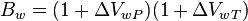$B_w = (1+\Delta V_{wP})(1+\Delta V_{wT})$

where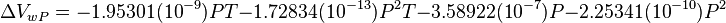$\Delta V_{wP} = -1.95301(10^{-9}) P T - 1.72834(10^{-13}) P^2 T - 3.58922(10^{-7}) P - 2.25341(10^{-10}) P^2$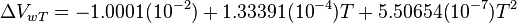$\Delta V_{wT} = -1.0001(10^{-2}) + 1.33391(10^{-4}) T + 5.50654(10^{-7}) T^2$

## Application range

The correlation is valid through the full range of solids concentrations, temperatures to 260F, and pressures to 5000psia.

## Example. Calculating water formation volume factor

Example source 

### Input data

Calculate water formation volume factor at 3176 psia and 165°F?

### Solution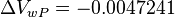$\Delta V_{wP} = -0.0047241$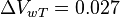$\Delta V_{wT} = 0.027$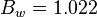$B_w = 1.022$

The solution is also available in the PVT software model at [The solution is available in the PVT calculator software model at www.pengtools.com

## Nomenclature$B_w$ = water formation volume factor, res bbl/STB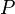$P$ = pressure, psia$T$ = Temperature, °F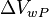$\Delta V_{wP}$ = pressure correction, res bbl/STB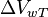$\Delta V_{wT}$ = temperature correction, res bbl/STB

## See also

Water bubble point pressure
Water compressibility
Water density
Water formation volume factor
Water salinity from density equation
Water solids concentration
Water viscosity
Gas/Water Interfacial Tension